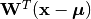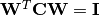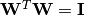Independent Component Analysis¶

Independent Component Analysis (ICA) is a computational technique for separating a multivariate signal into additive subcomponents, with the assumption that the subcomponents are non-Gaussian and independent from each other.

There are multiple algorithms for ICA. Currently, this package implements the Fast ICA algorithm.

ICA¶

The package uses a type ICA, defined below, to represent an ICA model:

mutable struct ICA{T<:Real}
mean::Vector{T}   # mean vector, of length m (or empty to indicate zero mean)
W::Matrix{T}      # component coefficient matrix, of size (m, k)
end

Note: Each column of W here corresponds to an independent component.

Several methods are provided to work with ICA. Let M be an instance of ICA:

indim(M)

Get the input dimension, i.e the number of observed mixtures.

outdim(M)

Get the output dimension, i.e the number of independent components.

mean(M)

Get the mean vector.

Note: if M.mean is empty, this function returns a zero vector of length indim(M).

transform(M, x)

Transform x to the output space to extract independent components, as.

Data Analysis¶

One can use fit to perform ICA over a given data set.

fit(ICA, X, k; ...)

Perform ICA over the data set given in X.

Parameters: X – The data matrix, of size (m, n). Each row corresponds to a mixed signal, while each column corresponds to an observation (e.g all signal value at a particular time step). k – The number of independent components to recover. The resultant ICA model, an instance of type ICA. Note: If do_whiten is true, the return W satisfies, otherwise W is orthonormal, i.eKeyword Arguments:

name description default
alg The choice of algorithm (must be :fastica) :fastica
fun

The approx neg-entropy functor. It can be obtained using the function icagfun. Now, it accepts the following values:

• icagfun(:tanh)
• icagfun(:tanh, a)
• icagfun(:gaus)
icagfun(:tanh)
do_whiten Whether to perform pre-whitening true
maxiter Maximum number of iterations 100
tol Tolerable change of W at convergence 1.0e-6
mean

The mean vector, which can be either of:

• 0: the input data has already been centralized
• nothing: this function will compute the mean
• a pre-computed mean vector
nothing
winit

Initial guess of W, which should be either of:

• empty matrix: the function will perform random initialization
• a matrix of size (k, k) (when do_whiten)
• a matrix of size (m, k) (when !do_whiten)
zeros(0,0)
verbose Whether to display iteration information false

Core Algorithms¶

The package also exports functions of the core algorithms. Sometimes, it can be more efficient to directly invoke them instead of going through the fit interface.

fastica!(W, X, fun, maxiter, tol, verbose)

Invoke the Fast ICA algorithm.

Parameters: W – The initial un-mixing matrix, of size (m, k). The function updates this matrix inplace. X – The data matrix, of size (m, n). This matrix is input only, and won’t be modified. fun – The approximate neg-entropy functor, which can be obtained using icagfun (see above). maxiter – Maximum number of iterations. tol – Tolerable change of W at convergence. verbose – Whether to display iteration information. The updated W.

Note: The number of components is inferred from W as size(W, 2).